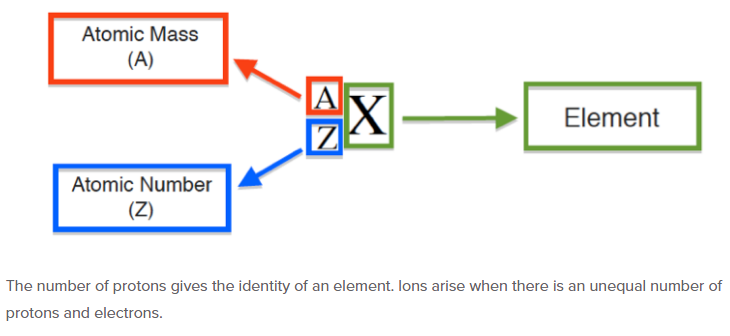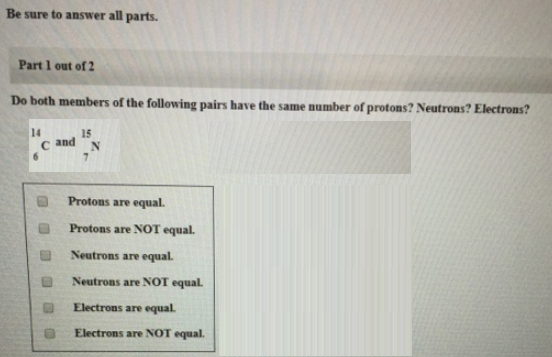# Problem: Be sure to answer all parts.Part 1 of 2Do both members of the following pairs have the same number of protons? Neutrons? Electrons? 14C6 and 15N7          (i) Protons are equal. (ii) Protons are NOT equal. (iii) Neutrons are equal. (iv) Neutrons are NOT equal. (v) Electrons are equal. (vi) Electrons are NOT equal.

🤓 Based on our data, we think this question is relevant for Professor Lin's class at VT.

###### FREE Expert Solution(i) Protons are equal. (False, atomic number = proton)###### Problem Details

Be sure to answer all parts.

Part 1 of 2

Do both members of the following pairs have the same number of protons? Neutrons? Electrons?

14C6 and 15N

(i) Protons are equal.

(ii) Protons are NOT equal.

(iii) Neutrons are equal.

(iv) Neutrons are NOT equal.

(v) Electrons are equal.

(vi) Electrons are NOT equal.